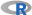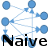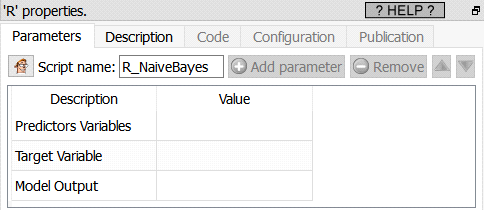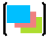﻿ 5. Detailed description of the Actions > 5.12. TA - R Predictive > 5.12.6. Naïve Bayes (R action)

# 5.12.6. Naïve Bayes (action)

Icon:Function: R_NaiveBayes

Property window:Short description:

Create a Naïve Bayes Model.

Long Description:

Naïve Bayes simply “smoothens” the dataset based on the conditional probabilities, basically computes the average probability of an event given a set of characteristics.

The Naïve Bayes algorithm is included in Anatella mainly because of historical reasons (and for explanatory/teaching purposes). Indeed, from a pratical point-of-view, the “Naïve Bayes” algorhtm is not very useful anymore because:

It’s impractical to use because the “Naïve Bayes” algorithm assumes that all your predictor variables are 100% un-correlated, which never happens in practice. Thus, before using theNaïve Bayes Action, you should first compute the Correlation Matrix (using theCovariance Action from section 5.7.11) to decide which variables you’ll keep inside your dataset (you must only keep un-correlated columns). This “cleaning” procedure is difficult and very time-consumming.

Compared to other modeling algorithms, the “Naïve Bayes” algorithm is not very accurate (other algorithms have typically higher AUC, and higher accuracy). This fact is visible in all datamining competitions (KDD cups, Kaggle) where the “Naïve Bayes” algorithm always ranks amongst the worst algorithms.

To use the Naïve Bayes action, simply select the predictors, target, and set the model output file name.